Image Restoration

Last updated on Monday, October 16, 1995 at 9:00 AM.

Gonzalez and Woods, 5.4.1 (deconvolution), 5.9.2 (gray-level interpolation)
These notes for Bayesian Reconstruction.

Deconvolution

We have already covered deconvolution as part of our discussion of filtering. Take a look at 5.4.1 an its example images for a demonstration of the technique.

Geometric Distortions

In addition to blurring and noise, other image distortions include geometric deformations. These deformations can often be corrected by applying warping transformations to the image.
When doing an image warping or other geometric transformation, it's often necessary to compute the intensity of the image at a point where no sample was taken (i.e., not on the pixel grid). To do so requires some form of interpolation between the samples.
Let's consider the simplest case of how to interpolate between uniformly spaced 2-D samples. One can use bilinearly interpolation as a simple way to smoothly interpolate between the pixels. Bilinear interpolation involves first interpolating vertically between the pixels on the left, then interpolating vertically between the pixels on the right, then interpolating horizontally between the first two interpolations.
While bilinear interpolation produces a smooth interpolation within pixels, it can produce sharp discontinuities in the first derivative across pixel boundaries. Smoother interpolation across pixels can be accomplished using higher-order interpolants such as bicubic interpolation.
When using image warps to correct geometric deformations, sometimes we need to interpolate between points that are not on a uniform grid. That is, the uniformly spaced samples of the pixel grid may really correspond to points that are not so uniformly spaced. We can still use bilinear or other forms of interpolation between the same set of points, but we just have to take into account their corrected positions and separations.

Bayesian Reconstruction

Formulation of the Problem

The general problem of reconstruction involves taking a given image g and, from it, attempting to deduce what the original scene f looked like. One form of reconstruction involves considering the space of all possible original scenes and selecting that which is the most probable given the image.
This probability can be written as the conditional probability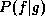. According to Bayes Theorem,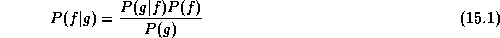This conditional probabilityis called the posterior probability.

The Data

The first factor in the denominator,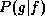, is the probability of getting image g as a result of imaging scene f. We can model this any way we want to, but the probability should be high when the image and the scene are similar--one doesn't expect to get an image that is too different from the original scene. (Imaging taking a picture of Marlon Brando and getting an image of Greta Garbo--that's more than we'd expect from noise, blurring, geometric distortions, etc.) Furthermore, it should model the known properties of the imaging system. One such function of modelling this probability is to use an inverse exponential function where the probabiity descreases as the difference between f and g increases.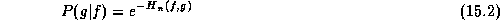If the image has Gaussian uncorrelated noise with standard deviation, we can measure this difference by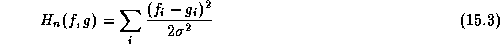In this case, the probabilityis precisely the mathematical probability of getting g given f.

The Prior

Notice, though, that the scene f that maximizes this probability is one identical to the image g. In other words, the best reconstruction is the original image. Obviously, this isn't very useful. This is where the other factor,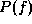, in the denominator comes in: the probability of any scene f occuring at all.is called the a priori probability: the probability of f being the scene without ever taking a picture g of it. This is called also called a prior.
In the prior, we take into account the desired properties that we want in the restored image. For example, we may assume that most scenes have patches of uniform intensity with sharp discontinuities between them. We can again describe the probability using an inverse exponential: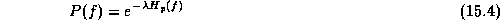where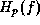measures the total of the differences between a pixels and their neighbors: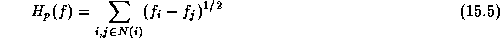Again, we can designto be whatever we want, so long as it embodies what we want in our reconstruction. A weight such asin Eq. 15.5 lets us control the relative priority of the prior.

The Image

The denomiator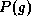measures the likelihood of any given image being produced by the imaging device, regardless of the input. If we assume that our device is equally capable of imaging any scene, this probability is constant for all g. Since our goal is simply to maximize the posterior probability, we don't really care what the exact value is, so long as we select the largest. For this reason, we can simply ignore the constant denominator.
If, however, we wanted to model tendencies for our device to produce images with certain properties, we could model it here.

The Optimization Goal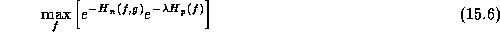The f that maximize this is the same one that maximizes the logarithm of the numerator: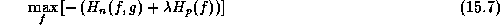Or, we could just as well minimize the negative of this quantity: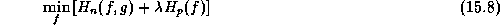This is simply the weighted sum of a data-fit term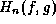and a prior term, where the weigthcontrols the relative importance.

Optimization Techniques

Clearly, the space of all possible scenes f is too large to search exhaustively. If we are reconstructing an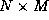image with L intensity levels, we have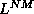possibilities!
Other optimization techniques have been developed that don't require exhaustive search.

If we're simply trying to find the lowest point (minimum), a simple approach is to start at some point that's hopefully close to the solution. From there, we simply head "downhill".
Remember that the gradient direction is the direction of steepest ascent. So, we head the other way. The basic algorithm is to select a start point, compute the gradient direction there, take a small step in the opposite direction, find the gradient there, and so on.
If we write the function to minimize as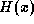, we can write the gradient descent algorithm as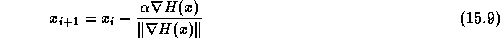wherecontrols how big a step we take. The selection ofis important--if it is too small we converge too slowly; it if is too large the method is unstable.
Eventually, we reach a minimum (where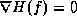) and stop.
The pitfall (pardon the pun) of gradient descent methods is that it goes downhill to the nearest relative minimum, not necessary the global minimum. If the function has only one minimum or if we start near enough to the global minimum, it works well; otherwise, it does not. If the image g isn't too far from the scene f, this is a reasonable approach.
Remember that each step in the iterative gradient descent algorithm is a potential scene (an image). If we watch the image at each step of the algorithm, we'll see it improve incrementally.

Simulated Annealing

Suppose that we use a gradient descent algorithm, but occasionally (with some probability) we allow it to go uphill. This means that for small dips in the function there is some chance that we'll climb out of it. Suppose that we further allow random jumps from one position to another, not every time but with some probability. The probability of these uphill moves and random jumps we'll control with a "temperature" parameter T that we'll diminish over time.
The result of this is somewhat like dropping a marble into a tray with some smoothly varying bottom. If we use only gradient descent, the marble will roll into the closes minimum. Adding some random component to the movement is like shaking the tray while the marble is rolling--most of the time the marble will roll downhill but every now and then it can bounce up and out of one valley and into another. Gradually, over time, we reduce the shaking until the ball settles comfortably into a minimum. Because of the shaking it's unlikely that this minimum is a shallow one--more likely that not it will settle into the deepest (or near deepest) minimum possible.
It has been shown that this solution will probabilistically find the global minimum. If we use an infinite cooling schedule and "shake" long enough, it has been proven that this will always find the global minimum.
This process is called simulated annealing, because it simulates the annealing (gradual cooling) process used for metals.

Similar Approaches

While this is just one approach, there are several other image processing or computer vision techniques that basically try to do the same thing. Even if not cast explicitly in terms of Bayesian probabily models, these models still try to simultaneously balance fidelity to the original image against assumptions of smoothness or other properties. Many of them use gradient descent techniques to iteratively smooth or otherwise restore the image.

Image Restoration as Segmentation

These image restoration techniques tend to produce images that are piecewise constant, smooth in most places with sharp breaks where necessary. For this reason, they're sometimes described as "thin membrane" or "thin plate" models.
More sophisticated models produce piecewise-linear rather than piecewise-constant regions. This allows for gradual changes across regions. Piecewise-constant smoothing tends to produce stairstepping in such areas of gradual transition.
Because the resulting restoration produces constant or linearly-varying regions, it also serves as a segmentation algorithm. (We'll get to more of these leter, but segmentation algorithms are those that divide up an image into pieces that hopefully correspond to objects in the scene.)

Vocabulary

• posterior probability
• prior probability